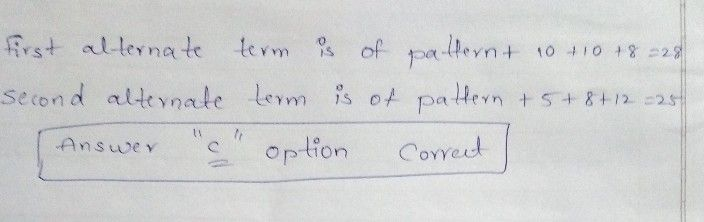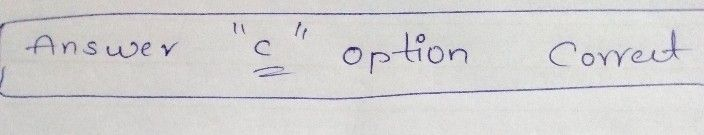Symbol
Problem11. $625,9,12.25,16,20.25,25,30.25,2$ $\left(A\right)$ $36$ $\left(B\right)32$ $\left(C\right)28.25$ $\left(D\right)40.25$
7th-9th grade
Other
SolutionQanda teacher - sathish